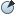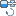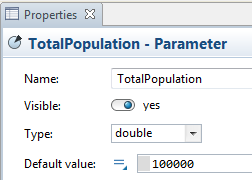AnyLogic

Now we will define constants of our model. In AnyLogic you define a constant by creating a parameter.

Define a constant representing total population

1. Drag theParameter element from theSystem Dynamics palette onto the graphical diagram of agent.
2. In the parameter’s Properties, set up the parameter properties.
3. Change the name of the constant. Type TotalPopulation in the Name edit box.
4. In the Default value edit box, type 100000. This will be the total population in our model.The rate, with which potential adopters come into contact with adopters, is assumed to be constant. So, we will define a constant to represent contact rate.

Define the ContactRate constant

1. Define the constant in the same way. Enter the Name: ContactRate.
2. Assume a contact rate of 100 per person per year. In the Default value, type 100.

In this model the volume of advertising and the probability that a potential adopter will adopt as the result of exposure to a given amount of advertising are assumed to be constant each period. So, we will add a constant to model the advertising effectiveness — the fractional adoption rate from advertising.

Define a constant representing advertising effectiveness

1. Define a constant in the similar way. Name it AdEffectiveness.
2. Set the value to 0.011.

Define one more constant to specify the adoption fraction — the proportion of contacts that are sufficiently persuasive to induce the potential adopter to purchase the product.